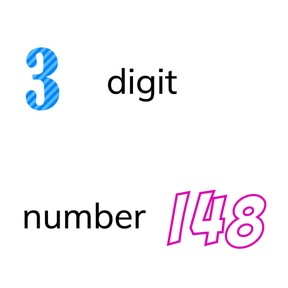Know the difference between a digit and a number >100

# Know the difference between a digit and a number >100

Know the difference between a digit and a number >100

No account needed.8,000 schools use Gynzy92,000 teachers use Gynzy1,600,000 students use Gynzy

## General

Students learn the difference between a digit (symbols 0-9) and numbers greater than 100, which are composed of a minimum of 3 digits.

2.NBT.A.1

## Relevance

It is important to know the difference between a digit and a number, furthermore it is also important to know how numbers greater than 100 are put together, namely that a number greater than 100 is made of a minimum of 3 digits.

## Introduction

Ask the students what three 1's is. Drag the cover away and reveal the joke- three 1's close together make 111.

## Development

Explain that digits are the symbols 0-9 and that numbers greater than 100 are always built using at least three digits. Next explain the pages with the hundreds, thousands, hundred-thousands, and millions showing students how they are built using more digits. Next ask the students to make a number greater than one hundred with the digits 9, 5, and 2. Next ask students to split the number 8317 into the separate digits it is made of. Ask a student to come to the front to create as big a number as possible with five digits. Ask students how many digits are in a prize of 2,500 dollars. The number has 4 digits, but the 0 is in the number twice, which means it only has 3 different digits.

Check that students understand the difference between a digit and a number by asking the following questions:
Are numbers and digits the same?
Can a number be made of digits or a digit made of numbers?
How many digits are in the number 4302?

## Guided Practice

Together with the students, look for digits in the given numbers, divide a thousands number into digits, and select the numbers that have three digits.

## Closing

Discuss the difference between digits and numbers and ask students if they can name a number greater than 100 and if their neighbour can say how many digits their number has. Next ask the students to make the largest possible, and the smallest number possible with the digits 2,5,8, and 1. First the students may only use the numbers once. On the next slide students may use the numbers as often as they like. They must make as many possible numbers with the given digits in one minute. The student who has come up with the most numbers wins.

### The online teaching platform for interactive whiteboards and displays in schools

• Save time building lessons

• Manage the classroom more efficiently

• Increase student engagement